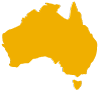###### madCAM 3X @ USD \$995 +GST
 madCAM 3X - \$1,502.11 AUD madCAM 3Xtra - \$2,860.81 AUD madCAM 4X - \$2,528.68 AUD madCAM 4Xtra - \$3,917.57 AUD madCAM 5X - \$4,521.44 AUD madCAM 5Xtra - \$9,050.42 AUD madCAM 3X - \$1,502.11 AUD madCAM 3Xtra - \$2,860.81 AUD madCAM 4X - \$2,528.68 AUD madCAM 4Xtra - \$3,917.57 AUD madCAM 5X - \$4,521.44 AUD madCAM 5Xtra - \$9,050.42 AUD For local currencypricing, please selectproduct option (left)AUD \$1502.11 + 10% GST =NZD \$1,625.29 + 15% GST =

madCAM provides the ability to generate 3, 4 and 5-axis toolpaths in Rhinoceros®. Rhino and madCAM also work superbly together as a CAM system, even when work is normally undertaken in another CAD system, because Rhino is extremely capable of importing files in other 3D formats.

## 2 1/2 axis Milling

3X 3Xtra 4X 4Xtra 5X 5Xtra
 Profiling X X X X X X Pocketing X X X X X X Facing X X X X X X Drilling X X X X X X Engraving X X X X X X V-carving X X X X X

## 3 axis Milling

3X 3Xtra 4X 4Xtra 5X 5Xtra
 Z-level Pocketing X X X X X X Z-level Facing X X X X X X Parallel Roughing X X X X X X Z-level finishing (Water line finishing) X X X X X X Planar finishing X X X X X X Planar contour finishing X X X X X Pencil Tracing X X X X X X Surface angle limit control (Steep or flat surface machining) X X X X X X Along Curves (Engraving) X X X X X X Project curves (Engraving) X X X X X X Between two curves X X X X X X From boundary curves X X X X X X Roughing from 3D Stock Model X X X X X Re-roughing X X X X Z-level remachining X X X X Planar remachining X X X X Contour remachining X X X X V-carving X X X X

## 4 axis Milling

3X 3Xtra 4X 4Xtra 5X 5Xtra
 Indexed 3 + 1-axis (Including all madCAM toolpath functions) X X X X Simultaneous 4-axis Profiling X X X X Simultaneous 4-axis Pocketing X X X X Simultaneous 4-axis Facing X X X X Simultaneous 4-axis Radial-level Pocketing X X X X Simultaneous 4-axis Radial-level Facing X X X X Simultaneous 4-axis Parallel Roughing X X X X Simultaneous 4-axis Radial-level finishing X X X X Simultaneous 4-axis Planar finishing X X X X Simultaneous 4-axis Planar contour finishing X X X X Simultaneous 4-axis Spiral finishing X X X X Simultaneous 4-axis Pencil Tracing X X X X Surface angle limit control (Steep or flat surface machining) X X X X Simultaneous 4-axis Along Curves (Engraving) X X X X Simultaneous 4-axis Project curves (Engraving) X X X X Simultaneous 4-axis Between two curves X X X X Simultaneous 4-axis From boundary curves X X X X Simultaneous 4-axis Roughing from 3D Stock Model X X X X Simultaneous 4-axis Radial-level remachining X X X Simultaneous 4-axis Planar remachining X X X Simultaneous 4-axis Contour remachining X X X Simultaneous 4-axis V-carving X X X

## 5 axis Milling

3X 3Xtra 4X 4Xtra 5X 5Xtra
 Indexed 3 + 2 axis (Including all madCAM toolpath functions) X X Simultaneous 5 axis from axis of revolution(Including all madCAM toolpath functions except re-roughing) X X Simultaneous 5-axis Drive Surface Offset-level Pocketing X Simultaneous 5-axis Drive Surface Offset-level Facing X Simultaneous 5-axis Drive Surface U-V Roughing X Simultaneous 5-axis Drive Surface Offset-level finishing X Simultaneous 5-axis Drive Surface U-V finishing X Simultaneous 5-axis Drive Surface border contour finishing X Simultaneous 5-axis Closed Drive Surface spiral finishing X Simultaneous 5-axis Drive Surface Pencil Tracing X Simultaneous 5-axis Drive Surface angle limit control X Simultaneous 5-axis SWARF Profiling from surface edge curves X Simultaneous 5-axis Profiling perpendicular to surface X Simultaneous 5-axis Part trimming from curves and vectors X

## Regions and Boundaries

3X 3Xtra 4X 4Xtra 5X 5Xtra
 Box work piece X X X X X X Region Curves X X X X X X Tool path Clipping planes X X X X X X User defined 3D stock models (Except simultaneous 5-axis drive surface) X X X X X Drive Surface calculation height and depth option X Regions from drive surface borders (trim curves) X Drive Surface tool path clipping levels option. X Simultaneous 5-axis vertical angle limit option X

## Toolpath editing

3X 3Xtra 4X 4Xtra 5X 5Xtra
 Transform and copy as ordinary Rhino curves (The toolpath curves are saved in Rhino as standard Rhino curves and can be transformed by using standard Rhino commands. It is also possible to edit control points on a toolpath curve.) X X X X X X

## Simulation

3X 3Xtra 4X 4Xtra 5X 5Xtra
 3-axis Material removal simulation X X X X X X 4-axis or 5-axis Material removal simulation X X X X Tool holder collision detection and simulation X X X X X X 4 axis machine parts simulation X X X X 5-axis machine parts simulation X X
 Custom machine 3X 3Xtra 4X 4Xtra 5X 5Xtra
 4-axis machine setup X X X X 5-axis machine setup X X Custom STL files support for machine parts simulation X X X X X External DLL support for machine kinematics X

## Post Processor

3X 3Xtra 4X 4Xtra 5X 5Xtra
 Customizable post processors X X X X X X 4-axis output X X X X 5-axis output X X 5-axis APT output X X Support for Inverse time feed (G93) X X X X

We Also Recommend